x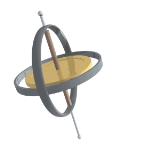PrecessionOverview

Precession is a change in the orientation of the rotation axis of a rotating
Rotation
A rotation is a circular movement of an object around a center of rotation. A three-dimensional object rotates always around an imaginary line called a rotation axis. If the axis is within the body, and passes through its center of mass the body is said to rotate upon itself, or spin. A rotation...

body. It can be defined as a change in direction of the rotation axis in which the second Euler angle
Euler angles
The Euler angles are three angles introduced by Leonhard Euler to describe the orientation of a rigid body. To describe such an orientation in 3-dimensional Euclidean space three parameters are required...

(nutation
Nutation
Nutation is a rocking, swaying, or nodding motion in the axis of rotation of a largely axially symmetric object, such as a gyroscope, planet, or bullet in flight, or as an intended behavior of a mechanism...

) is constant. In physics
Physics
Physics is a natural science that involves the study of matter and its motion through spacetime, along with related concepts such as energy and force. More broadly, it is the general analysis of nature, conducted in order to understand how the universe behaves.Physics is one of the oldest academic...

, there are two types of precession: torque
Torque
Torque, moment or moment of force , is the tendency of a force to rotate an object about an axis, fulcrum, or pivot. Just as a force is a push or a pull, a torque can be thought of as a twist....

-free and torque-induced.

In astronomy, "precession" refers to any of several slow changes in an astronomical body's rotational or orbital parameters, and especially to the Earth's precession of the equinoxes.Unanswered QuestionsEncyclopedia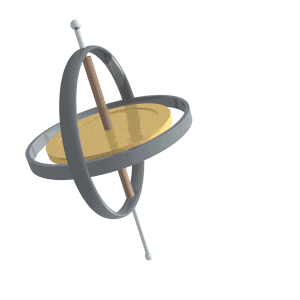Precession is a change in the orientation of the rotation axis of a rotating
Rotation
A rotation is a circular movement of an object around a center of rotation. A three-dimensional object rotates always around an imaginary line called a rotation axis. If the axis is within the body, and passes through its center of mass the body is said to rotate upon itself, or spin. A rotation...

body. It can be defined as a change in direction of the rotation axis in which the second Euler angle
Euler angles
The Euler angles are three angles introduced by Leonhard Euler to describe the orientation of a rigid body. To describe such an orientation in 3-dimensional Euclidean space three parameters are required...

(nutation
Nutation
Nutation is a rocking, swaying, or nodding motion in the axis of rotation of a largely axially symmetric object, such as a gyroscope, planet, or bullet in flight, or as an intended behavior of a mechanism...

) is constant. In physics
Physics
Physics is a natural science that involves the study of matter and its motion through spacetime, along with related concepts such as energy and force. More broadly, it is the general analysis of nature, conducted in order to understand how the universe behaves.Physics is one of the oldest academic...

, there are two types of precession: torque
Torque
Torque, moment or moment of force , is the tendency of a force to rotate an object about an axis, fulcrum, or pivot. Just as a force is a push or a pull, a torque can be thought of as a twist....

-free and torque-induced.

In astronomy, "precession" refers to any of several slow changes in an astronomical body's rotational or orbital parameters, and especially to the Earth's precession of the equinoxes. See Precession (astronomy).

## Torque-induced

Torque-induced precession (gyroscopic precession) is the phenomenon in which the axis of a spinning object (e.g., a part of a gyroscope
Gyroscope
A gyroscope is a device for measuring or maintaining orientation, based on the principles of angular momentum. In essence, a mechanical gyroscope is a spinning wheel or disk whose axle is free to take any orientation...

) "wobbles" when a torque
Torque
Torque, moment or moment of force , is the tendency of a force to rotate an object about an axis, fulcrum, or pivot. Just as a force is a push or a pull, a torque can be thought of as a twist....

is applied to it. The phenomenon is commonly seen in a spinning toy top, but all rotating objects can undergo precession. If the speed
Speed
In kinematics, the speed of an object is the magnitude of its velocity ; it is thus a scalar quantity. The average speed of an object in an interval of time is the distance traveled by the object divided by the duration of the interval; the instantaneous speed is the limit of the average speed as...

of the rotation and the magnitude
Magnitude (mathematics)
The magnitude of an object in mathematics is its size: a property by which it can be compared as larger or smaller than other objects of the same kind; in technical terms, an ordering of the class of objects to which it belongs....

of the torque are constant, the axis will describe a cone
Cone (geometry)
A cone is an n-dimensional geometric shape that tapers smoothly from a base to a point called the apex or vertex. Formally, it is the solid figure formed by the locus of all straight line segments that join the apex to the base...

, its movement at any instant being at right angle
Right angle
In geometry and trigonometry, a right angle is an angle that bisects the angle formed by two halves of a straight line. More precisely, if a ray is placed so that its endpoint is on a line and the adjacent angles are equal, then they are right angles...

s to the direction
Direction (geometry, geography)
Direction is the information contained in the relative position of one point with respect to another point without the distance information. Directions may be either relative to some indicated reference , or absolute according to some previously agreed upon frame of reference Direction is the...

of the torque. In the case of a toy top, if the axis is not perfectly vertical, the torque is applied by the force of gravity tending to tip it over.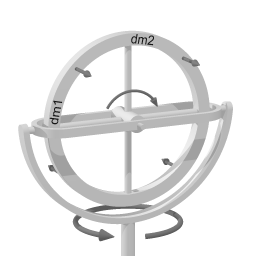The device depicted on the right here is gimbal
Gimbal
A gimbal is a pivoted support that allows the rotation of an object about a single axis. A set of two gimbals, one mounted on the other with pivot axes orthogonal, may be used to allow an object mounted on the innermost gimbal to remain immobile regardless of the motion of its support...

mounted. From inside to outside there are three axes of rotation: the hub of the wheel, the gimbal axis, and the vertical pivot.

To distinguish between the two horizontal axes, rotation around the wheel hub will be called 'spinning', and rotation around the gimbal axis will be called 'pitching.' Rotation around the vertical pivot axis is called 'rotation'.

First, imagine that the entire device is rotating around the (vertical) pivot axis. Then, spinning of the wheel (around the wheelhub) is added. Imagine the gimbal axis to be locked, so that the wheel cannot pitch. The gimbal axis has sensors, that measure whether there is a torque
Torque
Torque, moment or moment of force , is the tendency of a force to rotate an object about an axis, fulcrum, or pivot. Just as a force is a push or a pull, a torque can be thought of as a twist....

around the gimbal axis.

In the picture, a section of the wheel has been named dm1. At the depicted moment in time, section dm1 is at the perimeter
Perimeter
A perimeter is a path that surrounds an area. The word comes from the Greek peri and meter . The term may be used either for the path or its length - it can be thought of as the length of the outline of a shape. The perimeter of a circular area is called circumference.- Practical uses :Calculating...

of the rotating motion around the (vertical) pivot axis. Section dm1, therefore, has a lot of angular rotating velocity
Velocity
In physics, velocity is speed in a given direction. Speed describes only how fast an object is moving, whereas velocity gives both the speed and direction of the object's motion. To have a constant velocity, an object must have a constant speed and motion in a constant direction. Constant ...

with respect to the rotation around the pivot axis, and as dm1 is forced closer to the pivot axis of the rotation (by the wheel spinning further), due to the Coriolis effect
Coriolis effect
In physics, the Coriolis effect is a deflection of moving objects when they are viewed in a rotating reference frame. In a reference frame with clockwise rotation, the deflection is to the left of the motion of the object; in one with counter-clockwise rotation, the deflection is to the right...

dm1 tends to move in the direction of the top-left arrow in the diagram (shown at 45°) in the direction of rotation around the pivot axis. Section dm2 of the wheel starts out at the vertical pivot axis, and thus initially has zero angular rotating velocity with respect to the rotation around the pivot axis, before the wheel spins further. A force (again, a Coriolis force) would be required to increase section dm2's velocity up to the angular rotating velocity at the perimeter of the rotating motion around the pivot axis. If that force is not provided, then section dm2's inertia
Inertia
Inertia is the resistance of any physical object to a change in its state of motion or rest, or the tendency of an object to resist any change in its motion. It is proportional to an object's mass. The principle of inertia is one of the fundamental principles of classical physics which are used to...

will make it move in the direction of the top-right arrow. Note that both arrows point in the same direction.

The same reasoning applies for the bottom half of the wheel, but there the arrows point in the opposite direction to that of the top arrows. Combined over the entire wheel, there is a torque around the gimbal axis when some spinning is added to rotation around a vertical axis.

It is important to note that the torque around the gimbal axis arises without any delay; the response is instantaneous.

In the discussion above, the setup was kept unchanging by preventing pitching around the gimbal axis. In the case of a spinning toy top, when the spinning top starts tilting, gravity exerts a torque. However, instead of rolling over, the spinning top just pitches a little. This pitching motion reorients the spinning top with respect to the torque that is being exerted. The result is that the torque exerted by gravity - via the pitching motion - elicits gyroscopic precession (which in turn yields a counter torque against the gravity torque) rather than causing the spinning top to fall to its side.

Precession or gyroscopic considerations have an effect on bicycle
Bicycle
A bicycle, also known as a bike, pushbike or cycle, is a human-powered, pedal-driven, single-track vehicle, having two wheels attached to a frame, one behind the other. A person who rides a bicycle is called a cyclist, or bicyclist....

performance at high speed. Precession is also the mechanism behind gyrocompass
Gyrocompass
A gyrocompass­ is a type of non-magnetic compass which bases on a fast-spinning disc and rotation of our planet to automatically find geographical direction...

es.

Gyroscopic precession also plays a large role in the flight controls
Aircraft flight control systems
A conventional fixed-wing aircraft flight control system consists of flight control surfaces, the respective cockpit controls, connecting linkages, and the necessary operating mechanisms to control an aircraft's direction in flight...

on helicopter
Helicopter
A helicopter is a type of rotorcraft in which lift and thrust are supplied by one or more engine-driven rotors. This allows the helicopter to take off and land vertically, to hover, and to fly forwards, backwards, and laterally...

s. Since the driving force behind helicopters is the rotor disk
Helicopter rotor
A helicopter main rotor or rotor system is a type of fan that is used to generate both the aerodynamic lift force that supports the weight of the helicopter, and thrust which counteracts aerodynamic drag in forward flight...

(which rotates), gyroscopic precession comes into play. If the rotor disk is to be tilted forward (to gain forward velocity), its rotation requires that the downward net force
Net force
In physics, net force is the total force acting on an object. It is calculated by vector addition of all forces that are actually acting on that object. Net force has the same effect on the translational motion of the object as all actual forces taken together...

on the blade be applied roughly 90 degrees (depending on blade configuration) before, or when the blade is to one side of the pilot and rotating forward.

To ensure the pilot's inputs are correct, the aircraft has corrective linkages that vary the blade pitch in advance of the blade's position relative to the swashplate
Swashplate
A swashplate is a device used in mechanical engineering to translate the motion of a rotating shaft into reciprocating motion, or to translate a reciprocating motion into a rotating one to replace the crankshaft in engine designs.- Construction :...

. Although the swashplate moves in the intuitively correct direction, the blade pitch links are arranged to transmit the pitch in advance of the blade's position.

### Classical (Newtonian)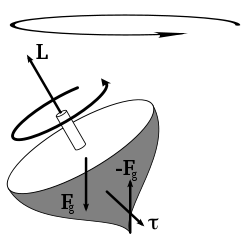Precession is the result of the angular velocity
Angular velocity
In physics, the angular velocity is a vector quantity which specifies the angular speed of an object and the axis about which the object is rotating. The SI unit of angular velocity is radians per second, although it may be measured in other units such as degrees per second, revolutions per...

of rotation and the angular velocity produced by the torque. It is an angular velocity about a line that makes an angle with the permanent rotation axis, and this angle lies in a plane
Angular momentum
In physics, angular momentum, moment of momentum, or rotational momentum is a conserved vector quantity that can be used to describe the overall state of a physical system...

at right angles to the plane of the couple producing the torque. The permanent axis must turn towards this line, since the body cannot continue to rotate about any line that is not a principal axis of maximum moment of inertia
Moment of inertia
In classical mechanics, moment of inertia, also called mass moment of inertia, rotational inertia, polar moment of inertia of mass, or the angular mass, is a measure of an object's resistance to changes to its rotation. It is the inertia of a rotating body with respect to its rotation...

; that is, the permanent axis turns in a direction at right angles to that in which the torque might be expected to turn it. If the rotating body is symmetrical
Symmetry
Symmetry generally conveys two primary meanings. The first is an imprecise sense of harmonious or aesthetically pleasing proportionality and balance; such that it reflects beauty or perfection...

and its motion unconstrained, and, if the torque on the spin axis is at right angles to that axis, the axis of precession will be perpendicular to both the spin axis and torque axis.

Under these circumstances the angular velocity of precession is given by:In which Is is the moment of inertia
Moment of inertia
In classical mechanics, moment of inertia, also called mass moment of inertia, rotational inertia, polar moment of inertia of mass, or the angular mass, is a measure of an object's resistance to changes to its rotation. It is the inertia of a rotating body with respect to its rotation...

,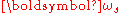is the angular velocity of spin about the spin axis, and m*g*r are the force and radius that comes from the torque. The torque vector originates at the center of mass. Using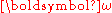=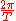, we find that the period
Frequency
Frequency is the number of occurrences of a repeating event per unit time. It is also referred to as temporal frequency.The period is the duration of one cycle in a repeating event, so the period is the reciprocal of the frequency...

of precession is given by: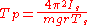In which Is is the moment of inertia
Moment of inertia
In classical mechanics, moment of inertia, also called mass moment of inertia, rotational inertia, polar moment of inertia of mass, or the angular mass, is a measure of an object's resistance to changes to its rotation. It is the inertia of a rotating body with respect to its rotation...

, Ts is the period of spin about the spin axis, andis the torque
Torque
Torque, moment or moment of force , is the tendency of a force to rotate an object about an axis, fulcrum, or pivot. Just as a force is a push or a pull, a torque can be thought of as a twist....

. In general, the problem is more complicated than this, however.

### Relativistic

The special and general theories of relativity
Theory of relativity
The theory of relativity, or simply relativity, encompasses two theories of Albert Einstein: special relativity and general relativity. However, the word relativity is sometimes used in reference to Galilean invariance....

give three types of corrections to the Newtonian precession, of a gyroscope near a large mass such as the earth, described above. They are:
• Thomas precession
Thomas precession
In physics the Thomas precession, named after Llewellyn Thomas, is a special relativistic correction that applies to the spin of an elementary particle or the rotation of a macroscopic gyroscope and relates the angular velocity of the spin of a particle following a curvilinear orbit to the angular...

a special relativistic correction accounting for the observer's being in a rotating non-inertial frame.
• de Sitter precession a general relativistic correction accounting for the schwarzschild metric of curved space near a large non-rotating mass.
• Lense-Thirring precession
Lense-Thirring precession
In general relativity, Lense–Thirring precession or the Lense–Thirring effect is a relativistic correction to the precession of a gyroscope near a large rotating mass such as the Earth. It is a gravitomagnetic frame-dragging effect...

a general relativistic correction accounting for the frame dragging by the Kerr metric of curved space near a large rotating mass.

## Astronomy

In astronomy
Astronomy
Astronomy is a natural science that deals with the study of celestial objects and phenomena that originate outside the atmosphere of Earth...

, precession refers to any of several gravity-induced, slow and continuous changes in an astronomical body's rotational axis or orbital path.

### Axial precession (precession of the equinoxes)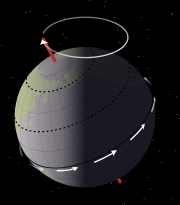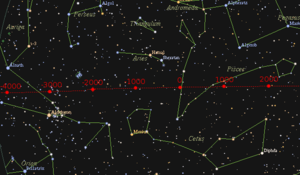Axial precession is the movement of the rotational axis of an astronomical body, whereby the axis slowly traces out a cone. In the case of Earth, this type of precession is also known as the precession of the equinoxes or precession of the equator. Earth goes through one such complete precessional cycle in a period of approximately 26,000 years, during which the positions of star
Star
A star is a massive, luminous sphere of plasma held together by gravity. At the end of its lifetime, a star can also contain a proportion of degenerate matter. The nearest star to Earth is the Sun, which is the source of most of the energy on Earth...

s as measured in the equatorial coordinate system
Equatorial coordinate system
The equatorial coordinate system is a widely-used method of mapping celestial objects. It functions by projecting the Earth's geographic poles and equator onto the celestial sphere. The projection of the Earth's equator onto the celestial sphere is called the celestial equator...

will slowly change; the change is due to the change of the coordinates. Over this cycle, Earth's north axial pole moves from where it is now, within 1° of Polaris
Polaris
Polaris |Alpha]] Ursae Minoris, commonly North Star or Pole Star, also Lodestar) is the brightest star in the constellation Ursa Minor. It is very close to the north celestial pole, making it the current northern pole star....

, in a circle around the ecliptic pole
Ecliptic pole
The ecliptic pole is the point on the celestial sphere where the sphere meets the imaginary line perpendicular to the ecliptic plane, the path the Earth travels on its orbit around the Sun.There are two ecliptic poles:...

, with an angular radius of about 23.5 degrees (or approximately 23 degrees 27 arcminutes ). The shift is 1 degree in 72 years, where the angle is taken from the observer, not from the center of the circle.

Hipparchus
Hipparchus
Hipparchus, the common Latinization of the Greek Hipparkhos, can mean:* Hipparchus, the ancient Greek astronomer** Hipparchic cycle, an astronomical cycle he created** Hipparchus , a lunar crater named in his honour...

is the earliest known astronomer to recognize and assess the precession of the equinoxes at almost 1º per century (which is not far from the actual value for antiquity, 1.38º). The Precession (axial rotation) was later explained by Newtonian physics
Classical mechanics
In physics, classical mechanics is one of the two major sub-fields of mechanics, which is concerned with the set of physical laws describing the motion of bodies under the action of a system of forces...

. Being an oblate spheroid, the Earth has a nonspherical shape, bulging outward at the equator. The gravitational tidal force
Tidal force
The tidal force is a secondary effect of the force of gravity and is responsible for the tides. It arises because the gravitational force per unit mass exerted on one body by a second body is not constant across its diameter, the side nearest to the second being more attracted by it than the side...

s of the Moon
Moon
The Moon is Earth's only known natural satellite,There are a number of near-Earth asteroids including 3753 Cruithne that are co-orbital with Earth: their orbits bring them close to Earth for periods of time but then alter in the long term . These are quasi-satellites and not true moons. For more...

and Sun
Sun
The Sun is the star at the center of the Solar System. It is almost perfectly spherical and consists of hot plasma interwoven with magnetic fields...

apply torque as they attempt to pull the equatorial bulge
Equatorial bulge
An equatorial bulge is a difference between the equatorial and polar diameters of a planet, due to the centrifugal force of its rotation. A rotating body tends to form an oblate spheroid rather than a sphere...

into the plane of the ecliptic
Ecliptic
The ecliptic is the plane of the earth's orbit around the sun. In more accurate terms, it is the intersection of the celestial sphere with the ecliptic plane, which is the geometric plane containing the mean orbit of the Earth around the Sun...

. The portion of the precession due to the combined action of the Sun and the Moon is called lunisolar precession.

### Perihelion precession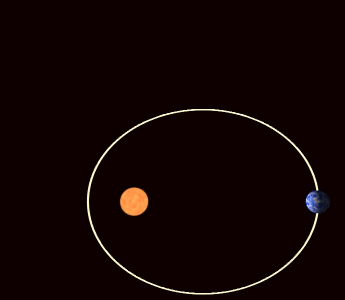The orbit
Orbit
In physics, an orbit is the gravitationally curved path of an object around a point in space, for example the orbit of a planet around the center of a star system, such as the Solar System...

of a planet around the Sun
Sun
The Sun is the star at the center of the Solar System. It is almost perfectly spherical and consists of hot plasma interwoven with magnetic fields...

is not really an ellipse but a flower-petal shape because the major axis of each planet's elliptical orbit also precesses within its orbital plane, partly in response to perturbations in the form of the changing gravitational forces exerted by other planets. This is called perihelion precession or apsidal precession
Apsidal precession
In celestial mechanics, perihelion precession, apsidal precession or orbital precession is the precession of the orbit of a celestial body. More precisely it is the gradual rotation of the line joining the apsides of an orbit, which are the points of closest and farthest approach...

.

Discrepancies between the observed perihelion precession rate of the planet Mercury
Mercury (planet)
Mercury is the innermost and smallest planet in the Solar System, orbiting the Sun once every 87.969 Earth days. The orbit of Mercury has the highest eccentricity of all the Solar System planets, and it has the smallest axial tilt. It completes three rotations about its axis for every two orbits...

and that predicted by classical mechanics
Classical mechanics
In physics, classical mechanics is one of the two major sub-fields of mechanics, which is concerned with the set of physical laws describing the motion of bodies under the action of a system of forces...

were prominent among the forms of experimental evidence leading to the acceptance of Einstein
Albert Einstein
Albert Einstein was a German-born theoretical physicist who developed the theory of general relativity, effecting a revolution in physics. For this achievement, Einstein is often regarded as the father of modern physics and one of the most prolific intellects in human history...

's Theory of Relativity
Theory of relativity
The theory of relativity, or simply relativity, encompasses two theories of Albert Einstein: special relativity and general relativity. However, the word relativity is sometimes used in reference to Galilean invariance....

(in particular, his General Theory of Relativity
General relativity
General relativity or the general theory of relativity is the geometric theory of gravitation published by Albert Einstein in 1916. It is the current description of gravitation in modern physics...

), which accurately predicted the anomalies.

These periodic changes of Earth's orbital parameters, combined with the precession of the equinoxes and of the inclination
Inclination
Inclination in general is the angle between a reference plane and another plane or axis of direction.-Orbits:The inclination is one of the six orbital parameters describing the shape and orientation of a celestial orbit...

of the Earth's axis on its orbit, are an important part of the astronomical theory
Milankovitch cycles
Milankovitch theory describes the collective effects of changes in the Earth's movements upon its climate, named after Serbian civil engineer and mathematician Milutin Milanković, who worked on it during First World War internment...

of ice age
Ice age
An ice age or, more precisely, glacial age, is a generic geological period of long-term reduction in the temperature of the Earth's surface and atmosphere, resulting in the presence or expansion of continental ice sheets, polar ice sheets and alpine glaciers...

s. See also nodal precession. For precession of the lunar orbit see lunar precession
Lunar precession
Precession is the rotation of a plane with respect to a reference plane. The orbit of the Moon has two important such precessional motions....

.

## See also

• De Sitter precession
Geodetic effect
The geodetic effect represents the effect of the curvature of spacetime, predicted by general relativity, on a vector carried along with an orbiting body...

• Larmor precession
Larmor precession
In physics, Larmor precession is the precession of the magnetic moments of electrons, atomic nuclei, and atoms about an external magnetic field...

• Lense–Thirring precession
• Nutation
Nutation
Nutation is a rocking, swaying, or nodding motion in the axis of rotation of a largely axially symmetric object, such as a gyroscope, planet, or bullet in flight, or as an intended behavior of a mechanism...

• Polar motion
Polar motion
Polar motion of the earth is the movement of Earth's rotational axis across its surface. This is measured with respect to a reference frame in which the solid Earth is fixed...

• Precession (mechanical)
Precession (mechanical)
Precession is the process of a round part in a round hole rotating with respect to that hole because of clearance between them and a radial force on the part that changes direction. The direction of rotation of the inner part is opposite to the direction of rotation of the radial force. Fretting...

• Thomas precession
Thomas precession
In physics the Thomas precession, named after Llewellyn Thomas, is a special relativistic correction that applies to the spin of an elementary particle or the rotation of a macroscopic gyroscope and relates the angular velocity of the spin of a particle following a curvilinear orbit to the angular...

• Euler angles
Euler angles
The Euler angles are three angles introduced by Leonhard Euler to describe the orientation of a rigid body. To describe such an orientation in 3-dimensional Euclidean space three parameters are required...

## External links

The source of this article is wikipedia, the free encyclopedia.  The text of this article is licensed under the GFDL.